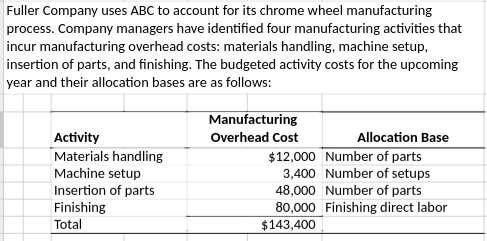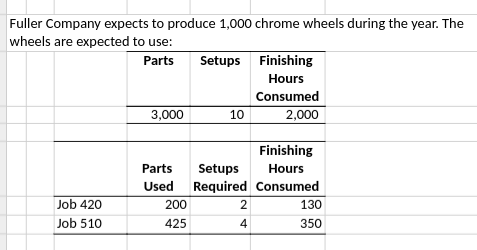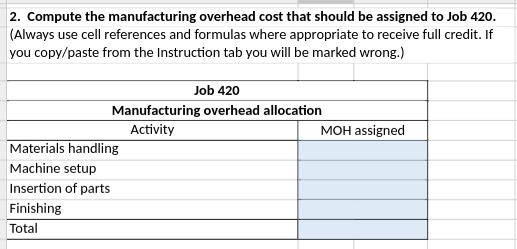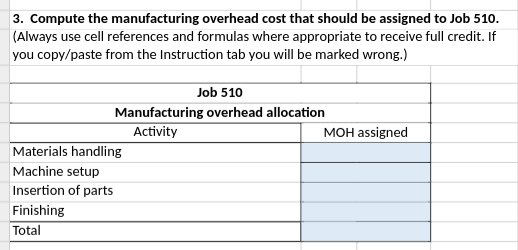In: Accounting

# fuller company uses abc to account for its chrome wheel manufacturing process## Solutions

##### Expert Solution

Requirement 1: Compute cost allocation rate for each activity

Formula:    $$\frac{\texttt{Manufacturing overheads}}{\texttt{Allocation base}}$$

 Manufacturing Overheads Allocation Base Activity Cost Allocation Rate Material handling $12,000 3000 parts$4 per part Machine Setups $3,400 10 setups$340 per setup Insertion of Parts $48,000 3000 parts$16 per part Finishing $80,000 2000 direct labor hours$40 per hour Total $143,400.00 Requirement 2: Formula:$${\texttt{Activity cost allocation rate}}\times {\texttt{Allocation base}}$$  Requirement 2: Compute manufacturing overhead cost for the JOB 420 Activity Activity Cost Allocation Rate Allocation base MOH Assigned Material handling$4 per part 200 Parts $800 Machine Setups$340 per setup 2 Setups $680 Insertion of Parts$16 per part 200 Parts $3,200 Finishing$40 per hour 130 direct labor hours $5,200 Total$9,880
 Requirement 3: Formula:$${\texttt{Activity cost allocation rate}}\times {\texttt{Allocation base}}$$   Compute manufacturing overhead cost for the JOB 510 Activity Cost Allocation Rate Allocation base MOH Assigned Material handling $4 per part 425 Parts$1,700 Machine Setups $340 per setup 4 Setups$1,360 Insertion of Parts $16 per part 425 Parts$6,800 Finishing $40 per hour 350 direct labor hours$14,000 Total $23,860 1. Cost allocation rate for each activity is calculated. 2. Manufacturing overhead cost assigned to the JOB 420 is$9880

3. Manufacturing overhead cost assigned to the JOB 420 is \$23860